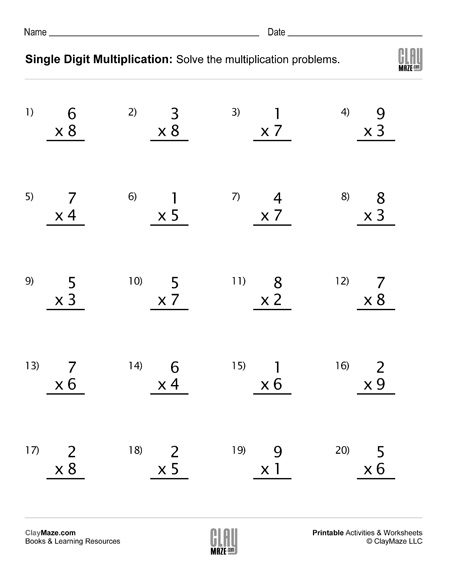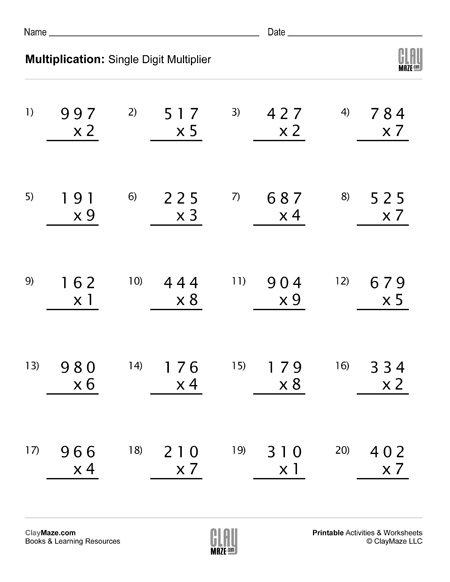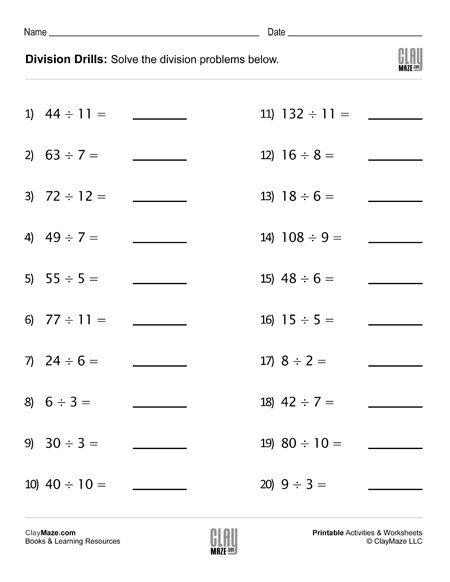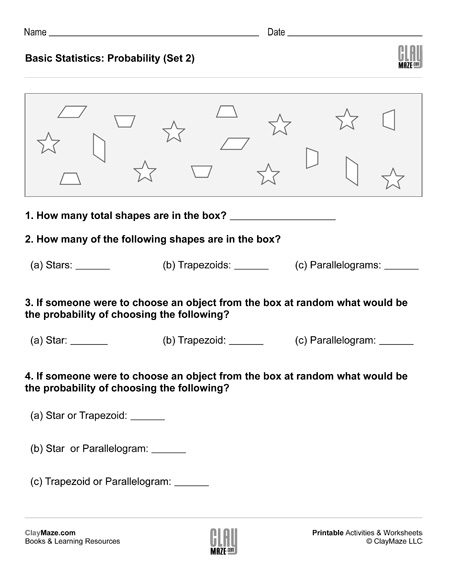### Free, Printable Worksheets and Resources

Great for the classroom or homeschool!
Our worksheets are completely free. – Share us with your friends!

• Print
• Learn

Welcome to ClayMaze.com!
Below is a random assortment of our hundreds of free children’s worksheets. Feel free to take a look around and browse our books or worksheets!

## Build Your Own Math Problems – Fun Paper Cut OutsThis is a great rainy day activity sheet. This math learning project is a little more interactive in that the child gets to cut out the squares necessary to build the problems and then can either a...

## Addition Worksheet (set-A): 3 digit addition – no regroupingFeel free to download our printable 3-digit addition worksheet – without carrying....

## Subtraction Worksheet – Fill in the Blanks Subtraction Facts (Set 2)Fill in the blank subtraction worksheet. 20 problems. – with solutions...

## Geometry: Find the Missing Angle in the Triangle (Set 2)Download this worksheet on finding the missing angle in a triangle. This worksheet assume the student is familiar with right, isosceles and scalene triangles and knows that the angles in a...

## Single Digit Multiplication Worksheet (Set 3)Single digit multiplication practice worksheet – 20 problems....

## Alphabet Trace and Write – lowercaseThis page is for letter writing practice. Trace the letters then write them in the space below the traced ones. Download and print this free worksheet. We also have free printable for uppercase...

## Multiplication with 3 Digits and Single Digit Multiplier (Set 4)20 problems – Multiplication practice worksheet – 3 digits with single digit multiplier – great for students beginning to learn multiplication....

## Find the Missing Addends Worksheet (Set 3)Download this worksheet on finding missing addends to reinforce  knowledge of addition and subtraction facts. – 20 problems...

## Blank CalendarDownload our blank calendar template for children to make their own calendars. It can also be used as a weekly schedule tool....

## Draw the Hands on the Analog Clocks (Set 2)Use this free worksheet for practice telling time with analog clocks. On this one, the student is asked to draw the hands on the clocks. Make sure they remember the hour hands moves slowly as the...

## Division Drills Worksheet (Set 3)Division Drills Worksheet with 20 division problems....

## Children’s Puzzle: Brain / Circle Shaped MazeEnjoy this brain / circle-shaped maze and remember that the second page of the PDF is the solution, so don’t peek! Holding a child’s visual attention can be a difficult thing. Their eyes and focus ...

## Subtraction Worksheet – Fill in the Blanks Subtraction Facts (Set 3)Worksheet for practice with subtraction facts. Fill in the blanks. Solutions included....

## Basic Statistics: Probability I (Set 2)This free worksheet covers basic probability. The student is asked several questions about the number of shapes in the box. The student can then use those values to find the probabilities of...

## Alphabetical Order with Word Bank – Topic: GeographyWrite the words from the word bank in alphabetical order. The topic of the words in this worksheet is geography....